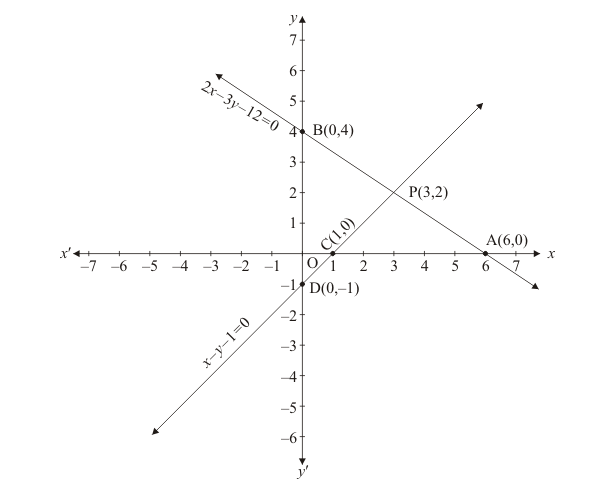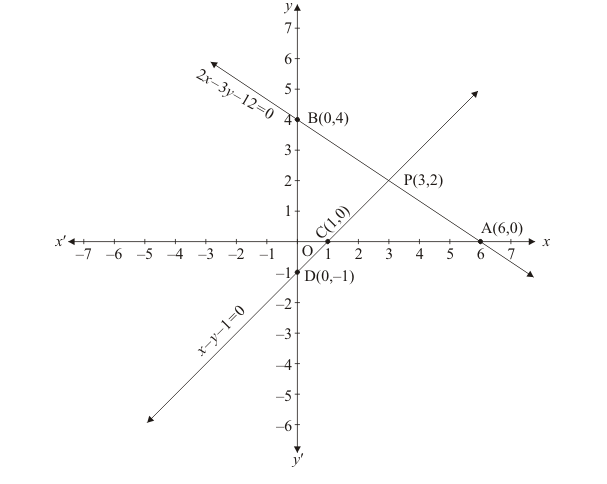# The area of the triangle formed by the lines`
Question:

The area of the triangle formed by the lines 2x + 3y = 12, x − y − 1 = 0 and x = 0 (as shown in Fig. 3.23), is(a) 7 sq. units

(b) $7.5 \mathrm{sq}$. units

(c) $6.5 \mathrm{sq}$. units

(d) 6 sq. units

Solution:

Given $2 x+3 y=12, x-y-1=0$ and $x=0$

If $x=0$ We have plotting points as $D(0,-1) B(0,4) P(3,2)$Therefore, area of $c B P D=\frac{1}{2}($ Base $\times$ Height $)=\frac{1}{2}(B P \times P M)=\frac{1}{2}(5 \times 3)=\frac{1}{2}(15)=7.5$

Area of triangle $A B C$ is $7.5$ square units

Hence, the correct choice is b